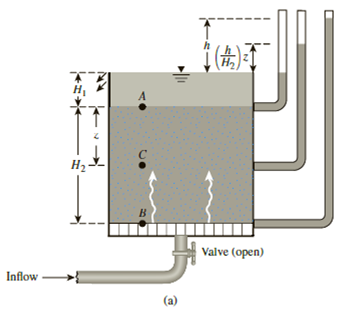Chapter 9, Problem 9.12PPrinciples of Geotechnical Enginee...

9th Edition
Braja M. Das + 1 other
ISBN: 9781305970939

Solutions

Chapter
SectionPrinciples of Geotechnical Enginee...

9th Edition
Braja M. Das + 1 other
ISBN: 9781305970939
Textbook Problem

Refer to Figure 9.4a. If H1 = 3 ft, H2 = 4.5 ft, h = 1.5 ft, γsat = 119 lb/ft3, area of the tank = 6.2 ft2, and hydraulic conductivity of the sand (k) = 0.31 ft/min,a. What is the rate of upward seepage of water (ft3/min)?b. If the point C is located at the middle of the soil layer, then what is the effective stress at C?(a)

To determine

Find the rate of upward seepage of water.

Explanation

Given information:

The depth of point A is H1 is 3 ft.

The depth between point A and B is H2 is 4.5 ft.

The depth (h) is 1.5 ft.

The area (A) of the tank is 6.2ft2.

The saturated unit weight of sand (γsat) is 119lb/ft3.

The hydraulic conductivity (k) of the soil is 0.31 ft/min.

Calculation:

Determine the hydraulic gradient i using the relation.

i=hH2

Substitute 1.5 ft for h and 4.5 ft for H2.

i=1

(b)

To determine

Find the effective stress σc at point C if the point C is located at the middle of the soil layer.

Still sussing out bartleby?

Check out a sample textbook solution.

See a sample solution

The Solution to Your Study Problems

Bartleby provides explanations to thousands of textbook problems written by our experts, many with advanced degrees!

Get Started

Why is important to know integral calculus?

Engineering Fundamentals: An Introduction to Engineering (MindTap Course List)

What are two ways to provide input for the speedometer?

Automotive Technology: A Systems Approach (MindTap Course List)

Compute the tension TAE in Sample Problem 5.7 using one scalar equilibrium equation.

International Edition---engineering Mechanics: Statics, 4th Edition

Explain the issues surrounding companies using customer data.

Enhanced Discovering Computers 2017 (Shelly Cashman Series) (MindTap Course List)